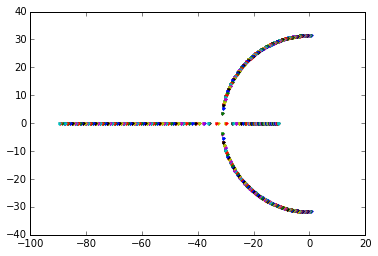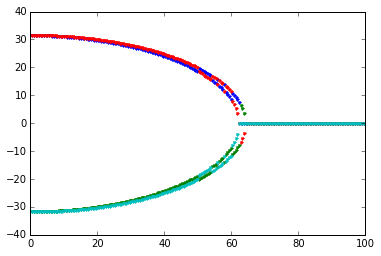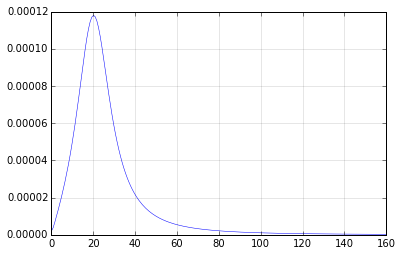## Exercise for the course Python for MATLAB users¶

Original exercise by Claus Führer, modified by Olivier Verdier

In :
%pylab
%matplotlib inline

Using matplotlib backend: MacOSX
Populating the interactive namespace from numpy and matplotlib


## System matrix¶

Consider the matrix $$A=\begin{bmatrix} 0 & I \\ K & D \end{bmatrix}$$ where $0$ and $I$ are the $2 \times 2$ zero and identity matrices and $K$ and $D$ are $2 \times 2$ matrices of the following form: $$K=\begin{bmatrix} -k & 0.5 \\ 0.5 & -k \end{bmatrix} \qquad D=\begin{bmatrix} -d & 1.0 \\ 1.0 & -d \end{bmatrix}$$ with $k$ and $d$ being real parameters.

### Write a function stiffness which constructs the matrix $K$ above.¶

In :
def make_sym(k,a):
"""Make a matrix of the form K or D"""
M = -k*identity(2)
M[0,1] = a
M[1,0] = a
return M

In :
def stiffness(k):
return make_sym(k,.5)

In :
assert(allclose(stiffness(1.), array([[-1.,.5],[.5,-1.]])))


### Write a function damping which constructs the matrix $D$ above.¶

In :
def damping(d):
return make_sym(d,1.)
#return zeros([2,2]) # implement this!

In :
assert(allclose(damping(1.), array([[-1.,1.],[1.,-1.]])))


### Write a function system_matrix which takes $k$ and $d$ as input and which generates the matrix $A$.¶

Hint: use the function concatenate. Check its documentation by running:

In :
concatenate?


Using concatenate:

In :
def system_matrix(d,k):
left = concatenate([zeros((2,2)), stiffness(k)])
right = concatenate([identity(2), damping(d)])
return concatenate([left, right], axis=1)


Using inplace replacements:

In :
def system_matrix(d,k):
M = zeros((4,4))
M[2:,:2] = stiffness(k)
M[2:,2:] = damping(d)
M[:2,2:] = identity(2)
return M


Use also identity (or eye), zeros (or zeros_like).

In :
A = system_matrix(10.,20.)
print(A)
assert(allclose(A[:2,:2], zeros([2,2])))
assert(allclose(A[:2,2:4], identity(2)))
assert(allclose(A[2:4,:2], stiffness(20.)))
assert(allclose(A[2:4,2:4], damping(10.)))

[[  0.    0.    1.    0. ]
[  0.    0.    0.    1. ]
[-20.    0.5 -10.    1. ]
[  0.5 -20.    1.  -10. ]]


### For samples of the values $d \in [0,100]$ and the fixed value $k=1000$, plot the four eigenvalues on the complex plane.¶

In :
for d in linspace(0,100,200):
M = system_matrix(d, 1000)
eigs = eigvals(M)
plot(eigs.real,eigs.imag,'.')### Bonus question: there is a bifurcation in the diagram above. Can you find the bifurcation point programmatically?¶

First, collect the data:

In :
def find_bifurcation(k):
eigs = []
ds = linspace(0,100,200)
for d in ds:
M = system_matrix(d, k)
eigs.append(eigvals(M))
# make an array:
aeigs = array(eigs)
# let's plot the imaginary parts:
plot(ds,aeigs.imag,'.')
# compute the part where the imaginary part is close to zero:
# we need all eigenvalues to be real:
# at which coefficient to we switch from False to True?
# which d was that:
return ds[i]

find_bifurcation(1000)

Out:
64.321608040200999## Frequency Response Plot¶

In technical applications there occurs often linear systems of the form $$\dot x(t) = A x(t) + B u(t)$$ where $u$ is an given input signal. $x$ is called the state. From the state some quantities $y(t)$ can be measured, this is decribed by the equation $$y(t)=C x(t).$$ We assume here that the input signal is an harmonic oscillation $u(t)=\sin(\omega t)$ with a given frequency $\omega$ and amplitude one. Then, $y(t)$ is again a harmonic oscillation with the same frequency, but another amplitude. The amplitude depends on the frequency.

The relationship between the in- and out-amplitude is given by the formula $$\mathrm{amplitude}(\omega)=\\|(G(i\omega))\\|\quad\text{where} \quad G(i\omega)=C \cdot (i\omega I -A)^{-1} \cdot B$$ and $i$ is the imaginary unit.

In :
inv?

In :
norm?

In :
def amplitude(A, B, C, omega):
#pass
M = 1j*omega*identity(len(A)) - A
return norm(dot(C, dot(inv(M), B)))


Plot the amplitude versus omega, for $\omega \in [0, 160]$, with $A$ being the system matrix above with $d=20$ and $k=500$, and $$C=\begin{bmatrix} 1 & 0 & 0 & 0 \end{bmatrix} \qquad B=\begin{bmatrix}0 \\ 0 \\ 0\\ 1 \end{bmatrix} .$$

In :
C = zeros((1,4))
B = zeros((4,1))
C[0,0] = 1
B[-1,0] = 1
A = system_matrix(20, 500)
def get_amplitudes(omegas):
amplitudes = []
for omega in omegas:
amplitudes.append(amplitude(A, B, C, omega))
return amplitudes
omegas_ = linspace(0,160,200)
plot(omegas_, get_amplitudes(omegas_), lw=.5)
grid(lw=.5, ls='-', alpha=.2)Find out the relationship between $A$'s eigenvalues and the peak(s) in the figure.